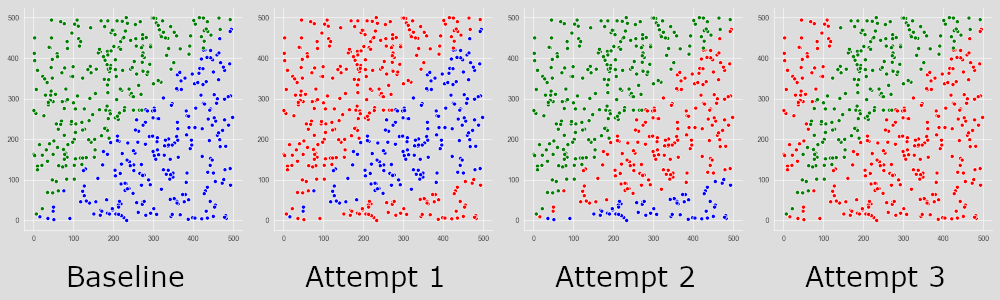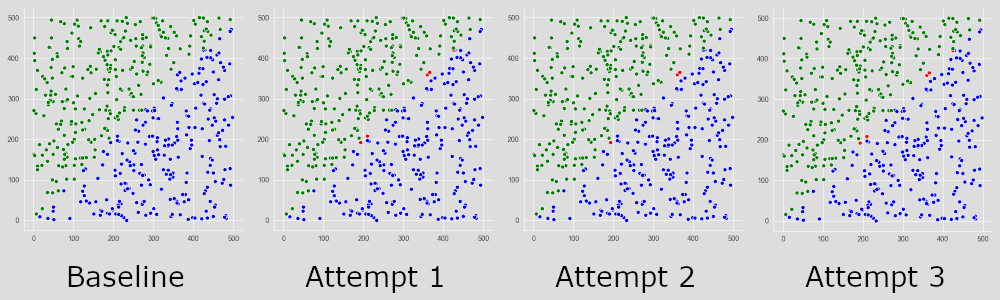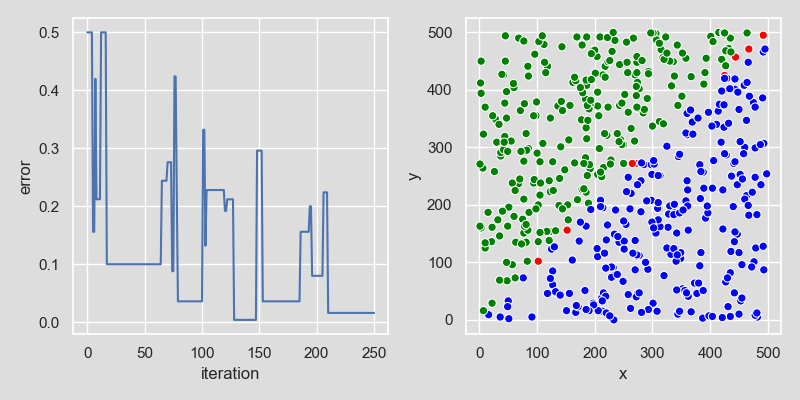Training our Neuron. Algorithms to keep it on track.

Part 2 of my foray into machine learning. In part one we built a neuron class in python. In this post we’ll start to train it to be useful… or as useful as a binary classifier can be.

If you haven’t read Part 1 I would highly suggest you start there. As a quick recap though, here is what we accomplished by the end of the last post.

We built a neuron class that looks like the following:

import random

class Neuron:
def __init__(self, number_of_inputs, weights=None):
self.number_of_inputs = number_of_inputs
self.weights = weights if weights is not None else self._random_weights()

def _random_weights(self):
return [random.normalvariate(0, .5) for _ in range(self.number_of_inputs)]

def _weighted_sum(self, inputs):
return sum([inputs[i] * self.weights[i] for i in range(self.number_of_inputs)])

def _activate(self, w_sum):
return -1 if w_sum < 0 else 1

def predict(self, inputs):
w_sum = self._weighted_sum(inputs)
return self._activate(w_sum)

We can instantiate it, and then get it to make predictions from inputs, but those predictions are basically a coin toss, based on the random weights assigned at initialization.

n = Neuron(number_of_inputs=2)
n.predict([100, 50])

Running this code a dozen times should yield a pretty random split of 1s and 0s. The goal of this post is to reduce that randomness it steer it closer and closer to a correct prediction.

Forward the Foundation

Baseline

To start, I created a bunch of random data for my perceptron to work with. Without doing any training, I ran it through several attempts at classifying the data. Here are a few of its outcomes:The green points are class A, the blue points are class b, and the red points were incorrectly guessed by the perceptron. Each of these attempts differs because the random starting weights between each perceptron were different. Attemp 2 is clearly on the right track, while the other two are very wrong. We’ll start steering these weights to get closer and closer to accurate predictions.

Step six: training

Our perceptron is going to go through supervised learning to train. This means we train it on a subset of data for which we know the expected outcome. Are the inputs above, class A or class B? Our neuron doesn’t know, so we need to tell it. It can then look at what it predicted, and what it should have predicted, and make some changes to itself to compensate. The only adjustments this neuron can make are to its weights, and we want it to be making adjustments based on its error. To calculate this error, we subtract the predicted value from the expected value, which gives us these possible error values:

| Desired | Predicted | Error |
|---------|-----------|-------|
|       1 |         1 |     0 |
|       1 |        -1 |     2 |
|      -1 |        -1 |     0 |
|      -1 |         1 |    -2 |

We then take these error values, multiply them by our training rate (I’ll come back to this), and then multiply that value by each input to get the values we need to steer each weight. I think this makes more sense to actually see in code, so here goes.

class Neuron:
# ...
for i in range(self.number_of_inputs):
self.weights[i] += inputs[i] * error * self.training_rate

So here, we take every input, multiply it by the overall error, then by the training rate, then we add that to that input’s respective weight.

Training Rate

Basically, a training rate is how hard you steer the neuron. Very high training rates will have a tendency to oscillate back and forth over the optimal weight, each time overshooting it, and trying to correct back, and subsequently overshooting again. To solve this, we steer by only small amounts at a time. This does mean it can take longer to land on the optimal weight. So there is a balance to how hard we are going to steer our weights, and that value will probably differ from dataset to dataset. I have chosen, somewhat arbitrarily, a training rate of 0.1, set at the neuron’s initialization.

class Neuron:
def __init__(self, number_of_inputs, weights=None, training_rate=0.1):
self.number_of_inputs = number_of_inputs
self.weights = weights if weights is not None else self._random_weights()
self.training_rate = training_rate

This makes it easy to play around with training rates without having to change my base neuron code each time. We have the ability to adjust our weights, now it’s time to flex those neuron muscles… brains have muscles, right? No… moving on…

Put in reps

We are going to make a function called, predictably, train. This function could work a couple of ways:

1. Take in a single set of inputs, the single expected output, make a prediction, and then adjust its weights, only doing this once per call.
2. Take in our whole set of training data, loop over it on its own, and train all at once.

For this neuron, I’m choosing to go with the second option, a step training process, feeding it the training data one piece at a time. This will make my implementation of my neuron slightly more cumbersome, since I’ll have to handle the data looping there, but it will allow me to do some interesting things with the neuron as a whole. Read on, you’ll see what I mean.

class Neuron:
# ...
def train(self, inputs, label):
output = self.predict(inputs)
error = label - output

Now that we can train, lets do it, and see what we get!As you can see here, with the same base data, and just a little bit of training, (I chose to train on only 50% of the data, so 250 data points) we can get very close to correct, even with each attempt having very different starting weights

To get these graphs, I actually handled the testing outside of the neuron class, by doing a for loop of my testing data and using the predict method, but we can easily have the neuron handle this.

Step 7: testing

While we are training one point at a time, I want to batch test, letting our neuron handle the looping.

class Neuron:
# ...
def test(self, x_test, y_test):
error = 0
for i, inputs in enumerate(x_test):
if self.predict(inputs) != y_test[i]:
error += 1
return error / len(x_test)

The x_test here is a 2D array of all the inputs, ie: x_test = [[10, 20], [75, 14], ...] and y_test is an array of all of the expected outputs, in the same order: y_test = [1, -1, ...]. So with this example: the expected output of [10, 20] would be 1, and [75, 14] would be -1.

Typically, training and testing data are split out from the same set of data, training on something like 70% of the data and testing on the unseen 30%. These percentages vary, and the process of splitting out the data is beyond the scope of this post.

The error rate we return here is just the average error percentage. So if we test on 250 data points and we get 5 of them wrong, our error rate would be 5 / 250 or 2%. I prefer to think in terms of accuracy, which would be 1 - error rate (98% here). But it seems pretty much all of machine learning talks about reducing error, rather than increasing accuracy, so I’ll stick with that.

Step Finally Done: tracking error rates

We have all of our parts in place, and now we can start to tie them together in interesting ways. The one I’m implementing is a simple way to track how training is affecting error rate. I’m calling this function run

class Neuron:
# ...
def run(self, x, y):
half = len(x) // 2
x_train = x[:half]
x_test = x[half:]
y_train = y[:half]
y_test = y[half:]
error_rates = [self.test(x_test, y_test)]
for i, inputs in enumerate(x_train):
self.train(inputs, y_train[i])
error_rates.append(self.test(x_test, y_test))
return error_rates

This function splits our data in half. We are assuming the data is sufficiently shuffled before coming in to this function. Data pre-processing is not really a part of building my neuron. Computerphile has a great playlist that goes in to this kind of thing much better than I could. See that here.

Now that we have split data, we grab see what our error rate is completely untrained. With our baseline in hand, we can start to see how training changes our error rates as we go. These should, ideally, trend towards 0. Let’s run it and find out!The plot on the left is the error rate over time, and the plot on the right is the final test result, after all the training has been done. Feel free to play with all this yourself. All of the code, plus some supplementary bits can be found here.

The whole enchilada. Thanks, now I want dinner!

Here it is. The whole kit and caboodle. Lock, stock, and barrel. Hook, line, and sinker. You get the idea…

import random

class Neuron:
def __init__(self, number_of_inputs, weights=None, training_rate=0.1):
self.number_of_inputs = number_of_inputs
self.weights = weights if weights is not None else self._random_weights()
self.training_rate = training_rate

def _random_weights(self):
return [random.normalvariate(0, .5) for _ in range(self.number_of_inputs)]

def _weighted_sum(self, inputs):
return sum([inputs[i] * self.weights[i] for i in range(self.number_of_inputs)])

def _activate(self, w_sum):
return -1 if w_sum < 0 else 1

for i in range(self.number_of_inputs):
self.weights[i] += inputs[i] * error * self.training_rate

def predict(self, inputs):
w_sum = self._weighted_sum(inputs)
return self._activate(w_sum)

def train(self, inputs, label):
output = self.predict(inputs)
error = label - output

def test(self, x_test, y_test):
error = 0
for i, inputs in x_test:
if self.predict(inputs) != y_test[i]:
error += 1
return error / len(x_test)

def run(self, x, y):
half = len(x) // 2
x_train = x[:half]
x_test = x[half:]
y_train = y[:half]
y_test = y[half:]
error_rates = [self.test(x_test, y_test)]
for i, inputs in enumerate(x_train):
self.train(inputs, y_train[i])
error_rates.append(self.test(x_test, y_test))
return error_rates

The core of this is just over 30 lines of code, and the whole thing is just under 50. Short, sweet, and to the point.

Stay tuned!

Part 3 of this series will be on a neural network, not just a perceptron.